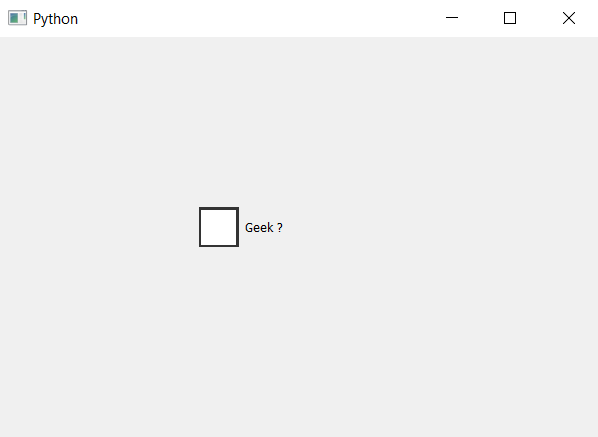Open In App

# PyQt5 – Changing size of Indicator in Check Box

In this article we will see how to change the size of indicator. Indicator is the part of check box although if we change the size of check box with the help of `resize` method or `setGeometry` method, but it will change the size of check box but not the indicator.

In order to change the size of indicator we have to change the height and width in the style sheet of the indicator which is used with check box object. Below is the style sheet code.

```QCheckBox::indicator
{
width : 40px;
height : 40px;
}
```

Below is the implementation.

 `# importing libraries``from` `PyQt5.QtWidgets ``import` `*` `from` `PyQt5 ``import` `QtCore, QtGui``from` `PyQt5.QtGui ``import` `*` `from` `PyQt5.QtCore ``import` `*` `import` `sys`` ` ` ` `class` `Window(QMainWindow):`` ` `    ``def` `__init__(``self``):``        ``super``().__init__()`` ` `        ``# setting title``        ``self``.setWindowTitle(``"Python "``)`` ` `        ``# setting geometry``        ``self``.setGeometry(``100``, ``100``, ``600``, ``400``)`` ` `        ``# calling method``        ``self``.UiComponents()`` ` `        ``# showing all the widgets``        ``self``.show()`` ` `    ``# method for widgets``    ``def` `UiComponents(``self``):`` ` `        ``# creating the check-box``        ``checkbox ``=` `QCheckBox(``'Geek ?'``, ``self``)`` ` `        ``# setting geometry of check box``        ``checkbox.setGeometry(``200``, ``150``, ``100``, ``80``)`` ` `        ``# setting stylesheet``        ``# changing width and height of indicator``        ``checkbox.setStyleSheet(``"QCheckBox::indicator"``                               ``"{"``                               ``"width :40px;"``                               ``"height : 40px;"``                               ``"}"``)`` ` ` ` ` ` `# create pyqt5 app``App ``=` `QApplication(sys.argv)`` ` `# create the instance of our Window``window ``=` `Window()`` ` `# start the app``sys.exit(App.``exec``())`

Output :